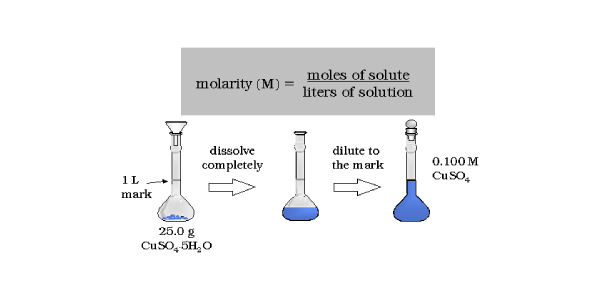# Chemistry Quiz On Molarity! Trivia

10 Questions | Attempts: 605
ShareSettingsDo you know what the term molarity means? M denotes molarity, and it is defined as the number of moles of solute present in one liter of solution. To obtain molarity, you will need to divide the moles of the solute by liters of solution. It is most expressed in units of moles. This chemistry quiz on molarity is top-notch. It would be best if you simply took this incredible quiz.

• 1.
How do you prepare a .3 M solution of NaCl? The molar mass of NaCl is 22.99 + 35.45. Since a .3 M solution is required,  multiply the molar mass by .3. Water is added to the 1-liter mark. REMEMBER: Molarity is Moles per Liter solution. The liter solution INCLUDES the water AND the salt -- salt has volume -- so it will be less than 1 Liter of water.
• A.

Find the molar mass of NaCl. Add that amount of NaCl to a volumetric flask and add distilled water to the 1 L mark. Stir.

• B.

Find the molar mass of NaCl. Add that amount of NaCl to a volumetric flask and add 1 Liter of distilled water. Stir.

• C.

Look up the molar mass of sodium and chlorine on the periodic table. Multiply the molar mass by .3. Place this amount in a volumetric flask and add distilled water to the 1 L mark. Stir.

• D.

Look up the molar mass of sodium and chlorine on the periodic table. Multiply the molar mass by .3. Place this amount in a volumetric flask and add 1 liter of distilled water. Stir.

• 2.
How much CaCl  and water is needed to make a .27 M solution?
• A.

29.96 g of Calcium chloride with enough distilled water to make 1 Liter.

• B.

110.98 g of Calcium chloride with enough water to equal 1 liter of solution.

• C.

29.96 g of Calcium chloride with 1 liter of distilled water.

• D.

110.98 g of Calcium chloride with 1 liter of distilled water.

• 3.
How much LiF and water are needed to make a .7 M solution?
• A.

Add 18.16 grams of LiF and 1 Liter of distilled water.

• B.

Add 25.94 grams of LiF and 1 Liter of distilled water.

• C.

Add 25.94 g of LiF to a volumetric flask with enough distilled water to reach the 1 Liter mark.

• D.

Add 18.16 grams of LiF with enough distilled water to make 1 Liter of solution.

• 4.
What is the molarity of a solution that contains 10.376 grams of LiF in a 250 ml solution?
• A.

1.6 M LiF solution

• B.

4 M LiF solution

• C.

1.2 M LiF solution

• D.

.4 Molar solution

• 5.
What is the molarity of a 300 mL solution containing 66.588 g of Calcium chloride?
• A.

0.002 M solution

• B.

2.0 M solution.

• C.

0.02 molar solution

• D.

20.0 molar solution

• 6.
What is the molarity of a solution containing 3.646 g of HCl (hydrochloric acid) in a 400 ml Solution?
• A.

1 M HCl solution

• B.

1.25 HCl solution

• C.

.25 M HCl Solution

• D.

0.025 M HCl Solution

• 7.
What is the molarity of a solution that contains 3 moles of Lithium Chloride in 2 Liters of the solution?
• A.

.66 M LiCl solution

• B.

1.5 M LiCl solution

• C.

6 M LiCl solution

• D.

2 M LiCl solution

• 8.
How is a 100 mL solution of .5 M HCl prepared from a stock of 6 M HCl solution? (Hint: This is dilution problem : (molarity of solution 1) (volume of solution 1) = (molarity of solution 2) (volume of solution 2). Dilution problems: You are solving for V1.  Set the equation: (6 M HCl) (Volume) = (.5 M HCl) (100 ml). IF  v2 is in ml, then v1 will also be in mL.
• A.

300 ml of a 6 M Solution

• B.

8.33 ml of a .5 M Solution

• C.

8.33 ml of a 3 M HCl solution

• D.

8.33 ml of 6 M HCl solution

• 9.
A teacher has a 12 M stock solution of HCl. Her students need 100 mL of .1 M solution to complete their lab. How is it prepared?
• A.

Add 8.3 mL of 12 M HCl to a graduated cylinder and add water to the 1 L mark.

• B.

Add 83 ml of 12 M HCl solution to volumetric flask and add water to the 1 Liter mark.

• C.

Take 0.83 mL of 12 M HCl and add it to a graduated cylinder; add distilled water to the 100 mL mark to get a .1 M solution.

• D.

Add 10 mL of 12 M HCl to a graduated cylinder and add water to the 100 ml mark.

• 10.
A teacher has a 12 M stock solution of HCl. Her students need 200 mL of 3.0 M solution to complete their lab. How is it prepared?
• A.

Add 50.0 mL of 12 M HCl to a graduated cylinder and add water to the 1 L mark.

• B.

Add 83 ml of 12 M HCl solution to volumetric flask and add water to the 1 Liter mark.

• C.

Take 5.0 of 12 M HCl and add it to a graduated cylinder; add distilled water to the 100 mL mark to get a .1 M solution.

• D.

Add 10 mL of 12 M HCl to a graduated cylinder and add water to the 100 ml mark.

## Related TopicsBack to top
×

Wait!
Here's an interesting quiz for you.